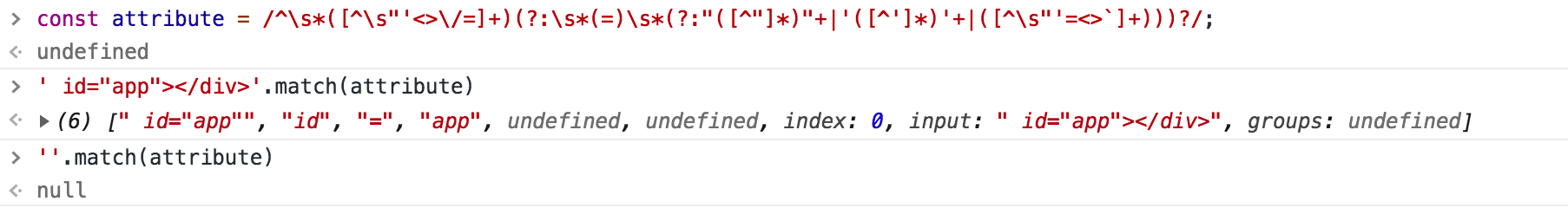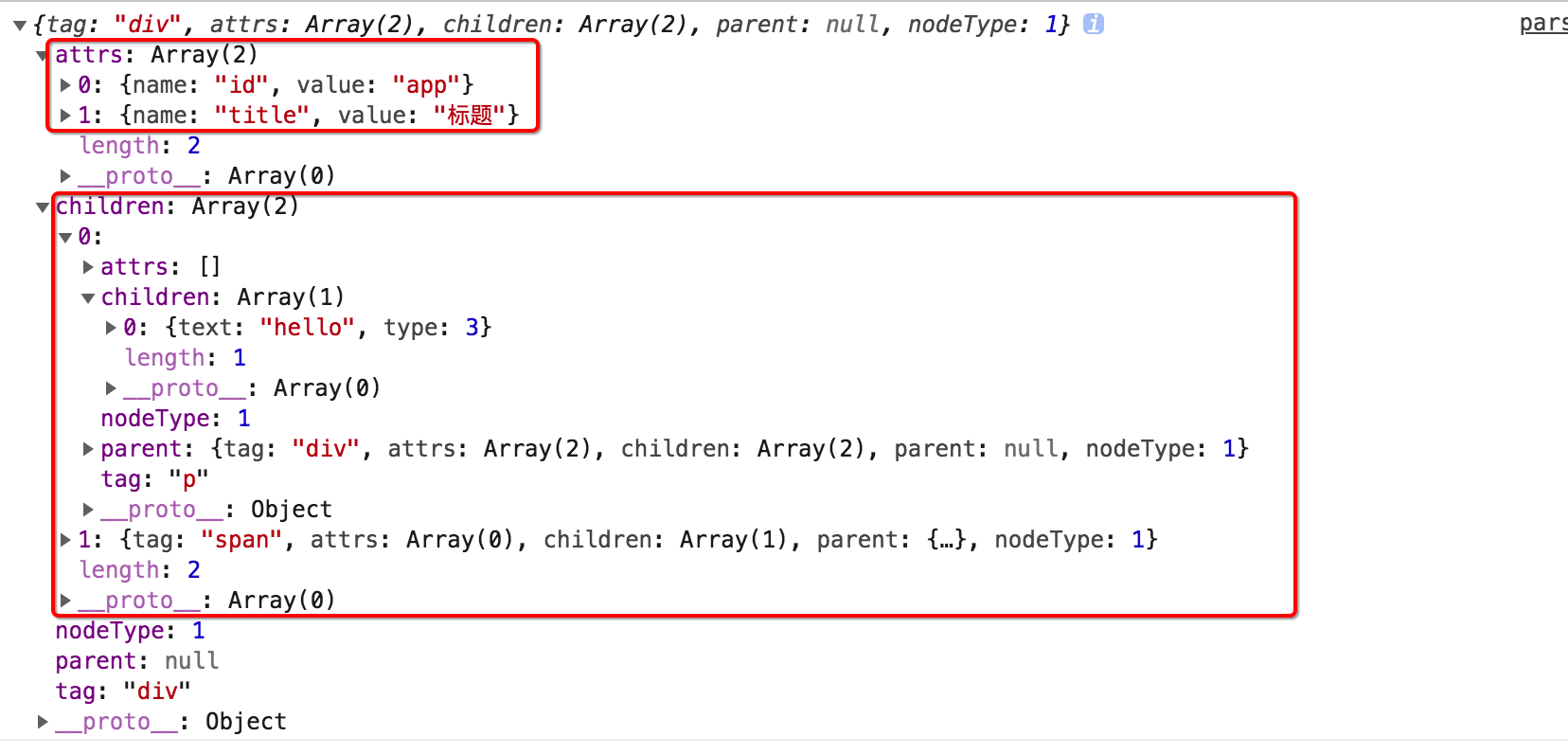# javaScript系列 -html-parser

``````<!-- 举例： -->
<div id="app"></div>
``````

``````{
tag: "div",
attrs:[{name:"id",value:"app"}],
}
``````

``````/* 形如：abc-123 */
const nc_name = `[a-zA-Z_][\\-\\.0-9_a-zA-Z]*`;

/* 形如：<aaa:bbb> */
const q_nameCapture = `((?:\${nc_name}\\:)?\${nc_name})`;

/* 形如：<div   匹配开始标签的左半部分 */
const startTagOpen = new RegExp(`^<\${q_nameCapture}`);

/* 匹配开始标签的右半部分(>) 形如`>`或者`  >`前面允许存在 N(N>=0)个空格 */
const startTagClose = /^\s*(\/?)>/;

/* 匹配属性节点：形如 id="app" 或者 id='app' 或者 id=app 等形式的字符串 */
const att =/^\s*([^\s"'<>\/=]+)(?:\s*(=)\s*(?:"([^"]*)"+|'([^']*)'+|([^\s"'=<div>`]+)))?/

let template = `<div id="app"></div>`;

function parser_html(html) {
/* 在字符串中搜索<字符并获取索引 */
let textStart = html.indexOf('<');

/* 标签的开头 */
if (textStart == 0) {
/* 匹配标签的开头 */
let start = html.match(startTagOpen);
/* start的结果为：["<div","div",...] */
if (start) {
const tagInfo = {
tag: start,
attrs: []
}

/* 删除已经匹配过的这部分标签 html->' id="app"></div>'*/
html = html.slice(start.length)

/* 匹配属性节点部分 */
/* 考虑到标签可能存在多个属性节点，因此这里使用循环 */
let attr, end;
/* 换言之：(如果 end 有值那么循环结束)，即当匹配到关闭标签的时候结束循环 */
while (!(end = html.match(startTagClose)) && (attr = html.match(att))) {
tagInfo.attrs.push({
name: attr,
value: attr || attr || attr
})
html = html.slice(attr.length)
}
/* html-> ' ></div>' */
if (end) {

/* 此处可能是'  >'因此第一个参数不能直接写0 */
html = html.slice(end.length);
/* html-> '</div>' */
/* 此处，关闭标签并不影响整体结果，因此暂不处理 */
return tagInfo;
}
}
}
}

let tree = parser_html(template);
console.log(tree);

/*

{ tag: 'div',
attrs: [ { name: 'id', value: 'app' } ] }
*/
console.log(parser_html(`<span id="app" title="标题"></span>`));
/*

{ tag: 'span',
attrs:
[ { name: 'id', value: 'app' }, { name: 'title', value: '标题' } ] }
*/
````````````/* 形如：abc-123 */
const nc_name = `[a-zA-Z_][\\-\\.0-9_a-zA-Z]*`;
/* 形如：<aaa:bbb> */
const q_nameCapture = `((?:\${nc_name}\\:)?\${nc_name})`;
/* 形如：<div   匹配开始标签的左半部分 */
const startTagOpen = new RegExp(`^<\${q_nameCapture}`);
/* 匹配开始标签的右半部分(>) 形如`>`或者`  >`前面允许存在 N(N>=0)个空格 */
const startTagClose = /^\s*(\/?)>/;
/* 匹配闭合标签：形如 </div> */
const endTag = new RegExp(`^<\\/\${q_nameCapture}[^>]*>`);
/* 匹配属性节点：形如 id="app" 或者 id='app' 或者 id=app 等形式的字符串 */
const att = /^\s*([^\s"'<>\/=]+)(?:\s*(=)\s*(?:"([^"]*)"+|'([^']*)'+|([^\s"'=<div>`]+)))?/

// const template = `<div><span class="span-class">Hi 夏!</span></div>`;
const template = `<div id="app" title="标题"><p>hello</p><span>vito</span></div>`

/* 标记节点类型(文本节点) */
let NODE_TYPE_TEXT = 3;
/* 标记节点类型(元素节点) */
let NODE_TYPE_ELEMENT = 1;

let stack = []; /* 数组模拟栈结构 */
let root = null;
let currentParent;

function compiler(html) {

/* 推进函数：每处理完一部分模板就向前推进删除一段 */
html = html.substring(n);
}

/* 解析开始标签部分：主要提取标签名和属性节点 */
function parser_start_html() {
/* 00-正则匹配 <div id="app" title="标题">模板结构*/
let start = html.match(startTagOpen);
if (start) {

/* 01-提取标签名称 形如 div */
const tagInfo = {
tag: start,
attrs: []
};

/* 删除<div部分 */

/* 02-提取属性节点部分 形如：id="app" title="标题"*/
let attr, end;
while (!(end = html.match(startTagClose)) && (attr = html.match(att))) {
tagInfo.attrs.push({
name: attr,
value: attr || attr || attr
});
}

/* 03-处理开始标签 形如 >*/
if (end) {
return tagInfo;
}
}
}

while (html) {
let textTag = html.indexOf('<');

/* 如果以<开头 */
if (textTag == 0) {
/* (1) 可能是开始标签 形如:<div id="app"> */
let startTagMatch = parser_start_html();
if (startTagMatch) {
start(startTagMatch.tag, startTagMatch.attrs);
continue;
}

/* (2) 可能是结束标签 形如:</div>*/
let endTagMatch = html.match(endTag);
if (endTagMatch) {
end(endTagMatch);
continue;
}
}

/* 文本内容的处理 */
let text;
if (textTag >= 0) {
text = html.substring(0, textTag);
}
if (text) {
chars(text);
}
}
return root;
}

/* 文本处理函数：<span>  hello <span> => text的值为 " hello "*/
function chars(text) {
/* 1.先处理文本字符串中所有的空格，全部替换为空 */
text = text.replace(/\s/g, '');

/* 2.把数据组织成{text:"hello",type:3}的形式保存为当前父节点的子元素 */
if (text) {
currentParent.children.push({
text,
type: NODE_TYPE_TEXT
})
}
}

function start(tag, attrs) {
let element = createASTElement(tag, attrs);
if (!root) {
root = element;
}
currentParent = element;
stack.push(element);
}

function end(tagName) {
let element = stack.pop();
currentParent = stack[stack.length - 1];
if (currentParent) {
element.parent = currentParent;
currentParent.children.push(element);
}
}

function createASTElement(tag, attrs) {
return {
tag,
attrs,
children: [],
parent: null,
nodeType: NODE_TYPE_ELEMENT
}
}

console.log(compiler(template));
``````### 推荐这些文章：

javaScript let/const定义

let/const定义

var定义，外部还能读取，但用let定义，外部不能访问内部，一般建议使用let
const常量定义,只读不能再次修改

<script>
'use strict'

function qj() {
...

leetcode原地删除系列-27

/**
<p>给定一个数组 <code>nums</code>，编写一个函数将所有 <code>0</code> 移动到数组的末尾，同时保持非零元素的相对顺序。</p>

<p><strong>请注意</strong>&n...

uni-app 布局固定头部，内容滚动

<template>
<view class="container">
<!-- toubu -->
<text>头部</text>
</view>
...

HTML的骨架标签

<html>
<title></title>
<body>

</body>
</html>

...

HTML标签元素常用列表

HTML 基本文档

<!DOCTYPE html>
<html>
<title>文档标题</title>
<body>

</body>
</html>

HTML: table表格标签横/纵向合并

Table横向合并

<table>
<tr>
<td width=100 colspan="2">位数/数值</td>
<td width=300>原码</td...

HTML 文本格式化标签

<!DOCTYPE html>
<html lang="en">
<meta charset="UTF-8">
<meta http-equiv="X-UA-Compatible" content="IE=edge">
<meta ...

HTML03：列表、表格标签和媒体元素

<!DOCTYPE html>

<html lang="en">

<meta charset="UTF-8">
<title>我的网页</title>

...

html iframe标签

iframe.html

<!DOCTYPE html>
<html>